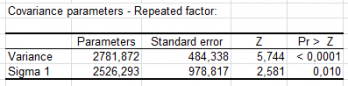# ANOVA mit wiederholten Messungen

Repeated measures ANOVA is an adaptation of ANOVA for cases where measures are repeated on the same statistical units. In Excel with the XLSTAT software.## Principles of Repeated measures Analysis of Variance

The principle of repeated measures ANOVA is simple. For each measure, a classical ANOVA model is estimated, then the sphericity of the covariance matrix between measures is tested using Mauchly’s test, Greenhouse-Geisser epsilon or Huynt-Feldt epsilon. If the sphericity hypothesis is not rejected, between- and within-subject effects can be tested.

## Calculation in Repeated measures Analysis of Variance

Repeated measures Analysis of Variance (ANOVA) uses the same conceptual framework as classical ANOVA. The main difference comes from the nature of the explanatory variables. The exploratory variable is measured at different time or repetition. In ANOVA, explanatory variables are often called factors.

If p is the number of factors, the ANOVA model is written as follows:

yti = β0 + ∑j=1...p βk(i,j),j + εi

where yti is the value observed for the dependent variable for observation i for measure t, k(i,j) is the index of the category of factor j for observation i, and εi is the error of the model.

The hypotheses used in ANOVA are identical to those used in linear regression: the errors εi follow the same normal distribution N(0,s) and are independent.

However, other hypotheses are necessary in the case of repeated measures ANOVA. As measures are taken from the same subjects at different times, the repetitions are correlated. In repeated measures ANOVA we assume that the covariance matrix between the ys is spherical (for example, compound symmetry is a spherical shape). We can drop this hypothesis when using the mixed model based approach.

XLSTAT can include in the model interactions and nested effects.

Several tests enable comparisons to be made between all pairs of categories and belong to the MCA test family (Multiple Comparisons of All, or All-Pairwise Comparisons):

• Tukey's HSD test,
• Bonferroni's t test,
• Dunn-Sidak's test,
• Newman-Keuls's test (SNK),
• Duncan's test,
• REGWQ test.

Enthalten ind